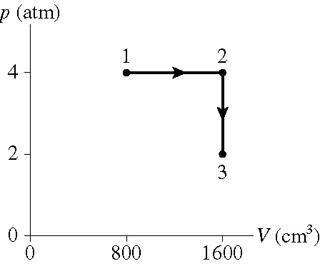# How much heat energy is transferred to or from the gas

0.250 mol of a monatomic gas follows the process shown in the figure.How much heat energy is transferred to or from the gas during process 1 to 2?

How much heat energy is transferred to or from the gas during process 2 to 3?

What is the total change in thermal energy of the gas?

I am not sure how to relate pressure and volume to heat energy.

I have $$pV=nRT$$ and $$\Delta E_{th}=W+Q$$

Also for the third question. would I just subtract the energy at point 3 from the energy at
point 1?

Last edited:

## Answers and Replies

Andrew Mason
Science Advisor
Homework Helper
Meteo said:
0.250 mol of a monatomic gas follows the process shown in the figure.How much heat energy is transferred to or from the gas during process 1 to 2?
How much heat energy is transferred to or from the gas during process 2 to 3?
What is the total change in thermal energy of the gas?
I am not sure how to relate pressure and volume to heat energy.
I have $$pV=nRT$$ and $$\DeltaE_th=W+Q$$
Also for the third question. would I just subtract the energy at point 3 from the energy at point 1?

The change in internal (thermal) energy of the gas is:

$$\Delta U = nC_v\Delta T = \Delta Q + W$$ where W is the work done to the gas (use -W if W is the work done by the gas).

What is $C_v$ for an ideal monatomic gas?

AM